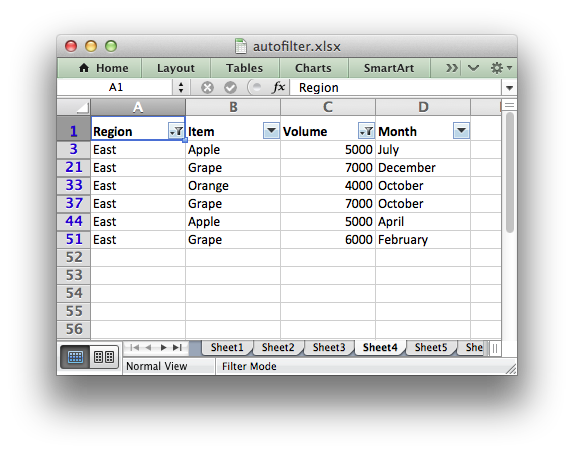# Example: Applying Autofilters

This program is an example of using autofilters in a worksheet. See Working with Autofilters for more details.```###############################################################################
#
# An example of how to create autofilters with XlsxWriter.
#
# An autofilter is a way of adding drop down lists to the headers of a 2D
# range of worksheet data. This allows users to filter the data based on
# simple criteria so that some data is shown and some is hidden.
#
# Copyright 2013-2023, John McNamara, jmcnamara@cpan.org
#
import xlsxwriter

workbook = xlsxwriter.Workbook("autofilter.xlsx")

# Add a worksheet for each autofilter example.

# Open a text file with autofilter example data.
textfile = open("autofilter_data.txt")

# Read the headers from the first line of the input file.

# Read the text file and store the field data.
data = []
for line in textfile:
# Split the input data based on whitespace.
row_data = line.strip("\n").split()

# Convert the number data from the text file.
for i, item in enumerate(row_data):
try:
row_data[i] = float(item)
except ValueError:
pass

data.append(row_data)

# Set up several sheets with the same data.
for worksheet in workbook.worksheets():
# Make the columns wider.
worksheet.set_column("A:D", 12)
# Make the header row larger.
worksheet.set_row(0, 20, bold)

###############################################################################
#
# Example 1. Autofilter without conditions.
#

# Set the autofilter.
worksheet1.autofilter("A1:D51")

row = 1
for row_data in data:
worksheet1.write_row(row, 0, row_data)

# Move on to the next worksheet row.
row += 1

###############################################################################
#
#
# Example 2. Autofilter with a filter condition in the first column.
#

# Autofilter range using Row-Column notation.
worksheet2.autofilter(0, 0, 50, 3)

# Add filter criteria. The placeholder "Region" in the filter is
# ignored and can be any string that adds clarity to the expression.
worksheet2.filter_column(0, "Region == East")

# Hide the rows that don't match the filter criteria.
row = 1
for row_data in data:
region = row_data

# Check for rows that match the filter.
if region == "East":
# Row matches the filter, no further action required.
pass
else:
# We need to hide rows that don't match the filter.
worksheet2.set_row(row, options={"hidden": True})

worksheet2.write_row(row, 0, row_data)

# Move on to the next worksheet row.
row += 1

###############################################################################
#
#
# Example 3. Autofilter with a dual filter condition in one of the columns.
#

# Set the autofilter.
worksheet3.autofilter("A1:D51")

worksheet3.filter_column("A", "x == East or x == South")

# Hide the rows that don't match the filter criteria.
row = 1
for row_data in data:
region = row_data

# Check for rows that match the filter.
if region == "East" or region == "South":
# Row matches the filter, no further action required.
pass
else:
# We need to hide rows that don't match the filter.
worksheet3.set_row(row, options={"hidden": True})

worksheet3.write_row(row, 0, row_data)

# Move on to the next worksheet row.
row += 1

###############################################################################
#
#
# Example 4. Autofilter with filter conditions in two columns.
#

# Set the autofilter.
worksheet4.autofilter("A1:D51")

worksheet4.filter_column("A", "x == East")
worksheet4.filter_column("C", "x > 3000 and x < 8000")

# Hide the rows that don't match the filter criteria.
row = 1
for row_data in data:
region = row_data
volume = int(row_data)

# Check for rows that match the filter.
if region == "East" and volume > 3000 and volume < 8000:
# Row matches the filter, no further action required.
pass
else:
# We need to hide rows that don't match the filter.
worksheet4.set_row(row, options={"hidden": True})

worksheet4.write_row(row, 0, row_data)

# Move on to the next worksheet row.
row += 1

###############################################################################
#
#
# Example 5. Autofilter with a filter list condition in one of the columns.
#

# Set the autofilter.
worksheet5.autofilter("A1:D51")

worksheet5.filter_column_list("A", ["East", "North", "South"])

# Hide the rows that don't match the filter criteria.
row = 1
for row_data in data:
region = row_data

# Check for rows that match the filter.
if region == "East" or region == "North" or region == "South":
# Row matches the filter, no further action required.
pass
else:
# We need to hide rows that don't match the filter.
worksheet5.set_row(row, options={"hidden": True})

worksheet5.write_row(row, 0, row_data)

# Move on to the next worksheet row.
row += 1

###############################################################################
#
#
# Example 6. Autofilter with filter for blanks.
#
# Create a blank cell in our test data.

# Set the autofilter.
worksheet6.autofilter("A1:D51")

worksheet6.filter_column("A", "x == Blanks")

# Simulate a blank cell in the data.
data = ""

# Hide the rows that don't match the filter criteria.
row = 1
for row_data in data:
region = row_data

# Check for rows that match the filter.
if region == "":
# Row matches the filter, no further action required.
pass
else:
# We need to hide rows that don't match the filter.
worksheet6.set_row(row, options={"hidden": True})

worksheet6.write_row(row, 0, row_data)

# Move on to the next worksheet row.
row += 1

###############################################################################
#
#
# Example 7. Autofilter with filter for non-blanks.
#

# Set the autofilter.
worksheet7.autofilter("A1:D51")

worksheet7.filter_column("A", "x == NonBlanks")

# Hide the rows that don't match the filter criteria.
row = 1
for row_data in data:
region = row_data

# Check for rows that match the filter.
if region != "":
# Row matches the filter, no further action required.
pass
else:
# We need to hide rows that don't match the filter.
worksheet7.set_row(row, options={"hidden": True})

worksheet7.write_row(row, 0, row_data)

# Move on to the next worksheet row.
row += 1

workbook.close()
```